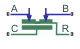# Cylinder Friction (IL)

Adds friction to cylinders with moving components

Since R2020a

•Libraries:
Simscape / Fluids / Isothermal Liquid / Actuators / Auxiliary Components

## Description

The Cylinder Friction (IL) block models friction on a moving cylinder in an isothermal liquid network. The total friction is a combination of the Stribeck, Coulomb, and viscous effects. The Coulomb friction component includes any initial force applied by the seal and the influence of pressure in the cylinder.

The Stribeck and viscous forces are calculated in the same way as for the Simscape Foundational Library Translational Friction block.

### The Stribeck Friction and Viscous Friction Forces

The Stribeck friction force, FStribeck, is the dominating friction component at low velocities. The block uses the equation

`${F}_{Stribeck}=\sqrt{2e}\left({R}_{break,Coulomb}-1\right)\cdot {F}_{C}\left(\frac{\upsilon }{{\upsilon }_{static,th}}\right){e}^{-{\left(\frac{\upsilon }{{\upsilon }_{static,th}}\right)}^{2}},$`

where:

• Rbreak,Coulomb is the value of the Breakaway to Coulomb friction force ratio parameter, ${R}_{Break,Coulomb}=\frac{{F}_{break}}{{F}_{Coulomb}}.$

• ν is the velocity of the cylinder, $\upsilon ={\upsilon }_{R}-{\upsilon }_{C}$.

• νstatic,th is the threshold velocity for static torque

`${\upsilon }_{static,th}=\sqrt{2}{v}_{break},$`

where vbreak is the value of the Breakaway friction velocity parameter. The block establishes a transition range between 0 and the threshold velocity for static torque to ensure smooth modeling of the friction force.

• FC is the Coulomb frictional force.

The viscous friction force is based on the value of the Viscous friction coefficient parameter for the working fluid, fviscous, and is proportional to the cylinder velocity

`${F}_{viscous}={f}_{viscous}\upsilon .$`

### The Coulomb Friction Force

The Coulomb friction force is a force that acts normal to the friction surface. The cylinder motion creates a radial stress inside the fixed cylinder casing, which increases as the cylinder compresses the internal fluid. The radial stress is normal to the cylinder motion, and results in a Coulomb friction force that opposes cylinder motion.

The Coulomb frictional force is

`${F}_{Coulomb}={F}_{c}\mathrm{tanh}\left(\frac{\upsilon }{{\upsilon }_{Coulomb,th}}\right),$`

where ν is the relative velocity between ports R and C, νRνC. νCoulomb,th is the threshold velocity for the Coulomb force,

`${\upsilon }_{Coulomb,th}=\frac{{v}_{break}}{10}.$`

The threshold velocity for the Coulomb force is a different quantity than the threshold velocity for the static force, which the block uses to calculate the Stribeck friction force, although they both act as a threshold region to ensure smooth modeling of the Coulomb and Stribeck forces.

FC is the force contribution from seal preloading and the pressure in the cylinder,

`${F}_{C}={F}_{preload}+{f}_{Coulomb}\left({P}_{A}+{P}_{B}\right),$`

where:

• fCoulomb is the value of the Coulomb friction force coefficient parameter.

• PA and PB are the pressures at cylinder ports A and B, respectively. These values are gauge pressures with respect to the environmental pressure. You can specify these values either as atmospheric or another user-defied value by using the Environment pressure specification parameter.

## Ports

### Conserving

expand all

Isothermal liquid conserving port associated with the liquid entry or exit point to the orifice.

Isothermal liquid conserving port associated with the liquid entry or exit point to the orifice.

Mechanical translational conserving port associated with the cylinder housing motion.

Mechanical translational conserving port associated with the cylinder rod motion.

## Parameters

expand all

Ratio of the breakaway force to the Coulomb friction force, ${R}_{Break,Coulomb}=\frac{{F}_{break}}{{F}_{Coulomb}}.$

Threshold for movement against friction force.

Initial force in the cylinder due to the seal assembly. The block uses this parameter to calculate the Coulomb friction force.

Coulomb friction force coefficient. The block uses this parameter to calculate the Coulomb friction force.

Viscous friction coefficient. This parameter is used to calculate the viscous friction force.

Pressure reference to use for the Coulomb friction calculation.

User-defined pressure reference for the Coulomb friction calculation.

#### Dependencies

To enable this parameter, set Environment pressure specification to ```Specified pressure```.

## Version History

Introduced in R2020a